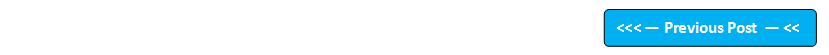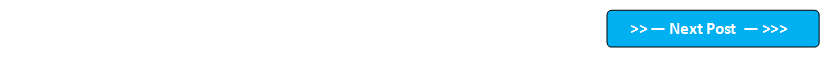## Blog Pages

### 273. static importWhen you import any class say 'ClassA' from a different package into your class say 'ClassB', you can now use all the methods of the imported 'ClassA' in your class 'ClassB'. But we have to use the following format to access the methods in our class 'ClassB' (i.e. Access imported class methods using class name) as shown in the below example  -

import package_path.ClassA;   //importing ClassA

public class ClassB
{

public static void main(String args[])
{

ClassA.method1( );   //Calling imported class methods using Class Name
ClassA.method2( );

}

}

But,we can access the members of the imported Class without using the Class Name. In order to do that, we have to update the above example according to the below two steps -

1. Add static keyword to the import statement
2. Add members to be imported, as the static import statements cannot import Classes, instead they import members of the Class as shown in the below -

import static package_path.ClassA; // Gives compiler error

import static package_path.ClassA.method1( );  //Wont give any compiler error, but we can only use method1( ) direclty

import static package_path.ClassA.*; //Can use all the methods of ClassA directly

Hence lets update the above code to access all methods of imported Class 'ClassA' directly (i.e. without using Class Name) as shown below -

import static package_path.ClassA.*;   //importing ClassA

public class ClassB
{

public static void main(String args[])
{

method1( );   //Calling imported class methods without using ClassA
method2( );

}

}

Lets implement this on Eclipse IDE (Access Math Class methods max( ) and min( ) directly)   -

1. Create a new Java Project 'Project 52' as shown below -

2. Create a new java class 'StaticImportDemo.java' with main( ) method as shown below -

3. Print the minimum and maximum values of two provided integers values by accessing the min( ) and math( ) methods using Math Class as shown below -

4. Save & Run the Java Program and observe that the first print statement has printed the max value i.e. 30 and second print statement has printed the min value i.e. 20 as shown below -

5. Now lets access the min( ) and max( ) methods directly without using the Class Math. In order to access them we have to add the following static import statement to the program -

6. View the error and select 'Change project compliance and JRE to 1.5' as shown below -

7. Observe that the error got resolved and now remove the class names before the methods as shown below -

8. Observe that the code is now changed as shown below -

9. Because of the static import statement, we are able to access all the methods (i.e. min( )  and max( ))of the Math Class directly in our code as shown above. Now lets Save & Run the program and observe that the max and min values are displayed in the output as shown below -

Hence by using static import statements, we can directly access the members of the imported classes (i.e. without using their class names).